# Moving Average in Microsoft Excel

Moving Average is an analytical tool in Microsoft Excel which is used to recognize the ongoing trend in the data and it helps in forecasting.

This tool is commonly used in several business to move an average of 3 months sales and present the report in excel chart

The Moving Average function is part of Add INS in Microsoft Excel 2010 and 2013.

To access this tool click on Data Tab, click on Data Analysis in the Analysis group. If the Data Analysis command is not available then you need to load the Analysis Toolpak.

How to Install Analysis ToolPak in Excel For Mac and Windows

Analysis toolpak of excel contains very helpful tools for statistical, financial, and engineering data analysis.

As the article suggests, today we will learn how to add Analysis ToolPak in Excel For Mac and Windows PCs. So lets get started.

#### How to Add Analysis ToolPak in Excel 2016 in Windows?

To install Analysis toolPak in Excel 2016 follow these steps.

1. Click on file.
2. Click on option. You’ll see excel option dialog box.
3. Click on Add-Ins. It is on the left, second option from bottom.
4. Locate Analysis ToolPack and click on it.
5. In the bottom, you must be able to see manage drop down. Select Excel-Add-ins and click on Go button.
6. All available Add-ins will be shown to you. Select Analysis Toolpak and click OK.

Analysis ToolPak is added now. You can see it in Data tab in the right most corner.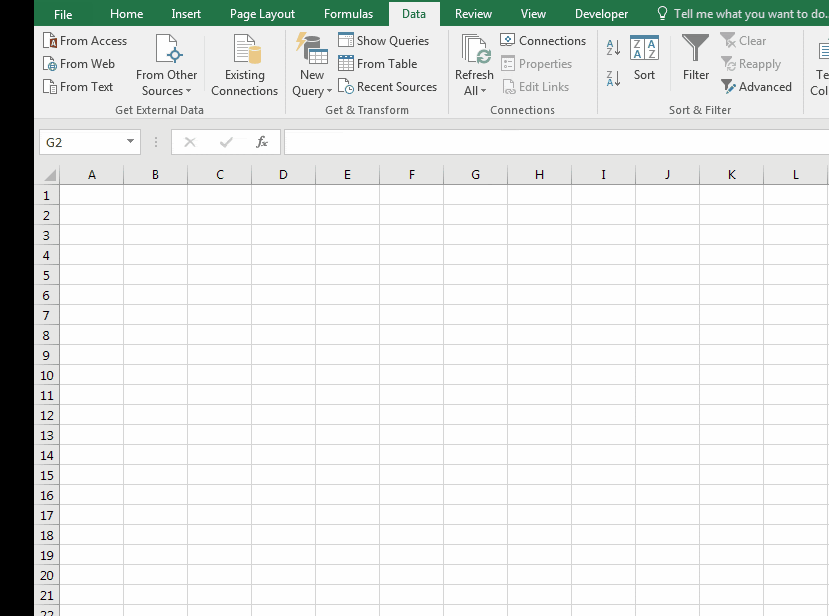#### How to Add Analysis ToolPak in Excel 2016 for Mac?

To install Analysis toolpak in Excel for Mac, follow below steps.

1. Goto tools or Insert menu.
2. Locate Add-Ins and Click on it.
3. You’ll be prompted to choose from available add-ins. Select Analysis Toolpak.
4.  Click Ok

Analysis Toolpak is added. Goto data tab. In the rightmost corner you’ll find Analysis Toolpak waiting for you.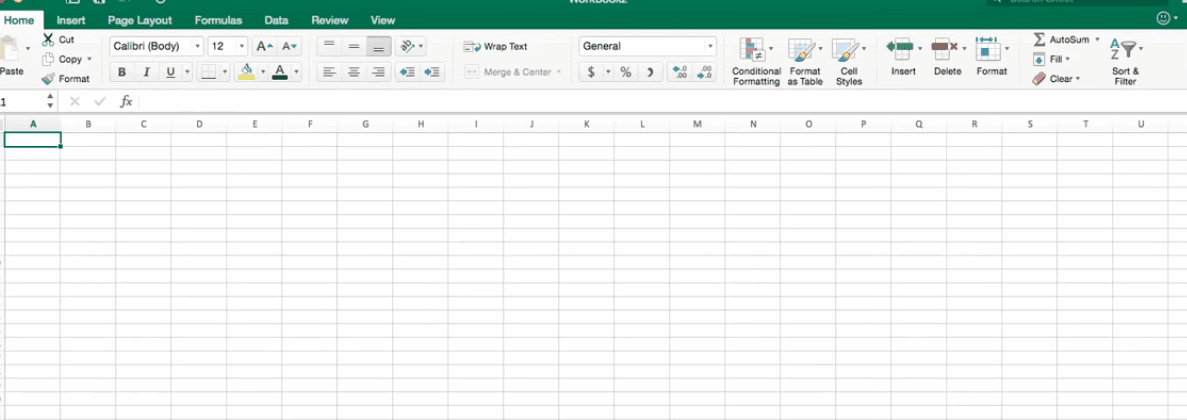Analysis Toolpak has a number of useful statistical tools that we will explore in our that we have explored in analysis tutorials.

Let’s come back on the topic, to generate the moving average report take an example. We have sales data month wise in the range A1:B13. In which column A contains Month name and column B sales.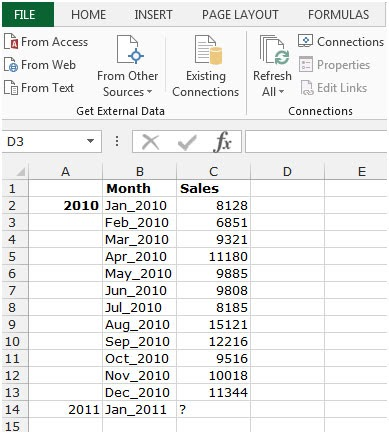To use theMoving Average tool, follow below mentioned steps:-

• Go to Data Tab.
• Click on Data Analysis in the Analyses group.
•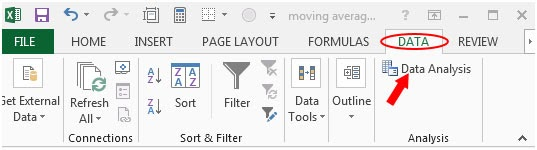• Data Analysis dialog box will appear.
• From the Analysis tool drop down menu select Moving Average and click on ok.
•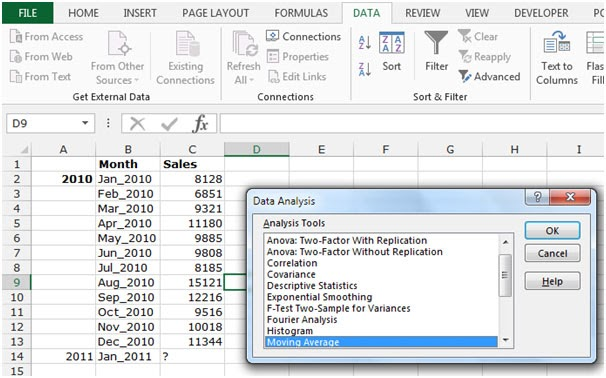• You will get another Moving Averagedialog box will appear.
• Click on Input range. Select the range C2:C13.
• Tick on Output Range and select the cell where you want to show the summary.
• Tick on the chart output in the box.
• Click on ok.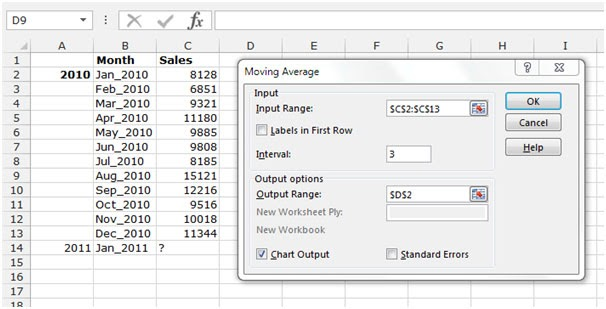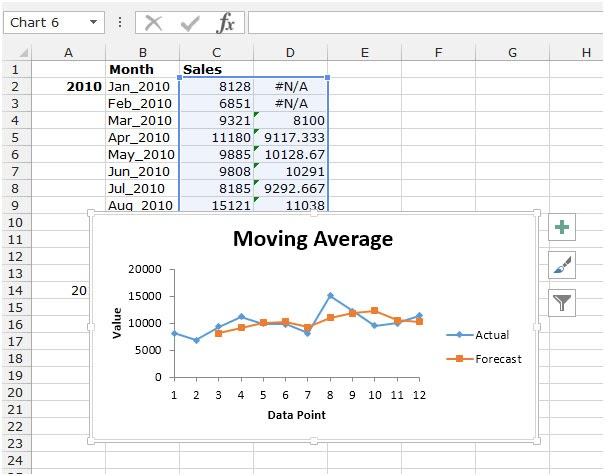We can see the sales performance trend in above snapshot in just one click by using the Moving Average tool in Microsoft Excel.

In this way, we learnt How to use the Moving Average in Microsoft Excel. You can use these functions in Excel 2016, 2013 and 2010. Find more articles on Mathematical formulation with different criteria. If you liked our blogs, share it with your friends on Facebook. And also you can follow us on Twitter and Facebook. We would love to hear from you, do let us know how we can improve, complement or innovate our work and make it better for you. Write us at info@exceltip.com

Related Articles

How To Highlight Cells Above and Below Average Value : highlight values which are above or below the average value using the conditional formatting in Excel.

Ignore zero in the Average of numbers : calculate the average of numbers in the array ignoring zeros using AVERAGEIF function in Excel.

Calculate Weighted Average : find the average of values having different weight using SUMPRODUCT function in Excel.

Average Difference between lists : calculate the difference in average of two different lists. Learn more about how to calculate average using basic mathematical average formula.

Average numbers if not blank in Excel : extract average of values if cell is not blank in excel.

AVERAGE of top 3 scores in a list in excel : Find the average of numbers with criteria as highest 3 numbers from the list in Excel

Popular Articles:

How to use the IF Function in Excel : The IF statement in Excel checks the condition and returns a specific value if the condition is TRUE or returns another specific value if FALSE.

How to use the VLOOKUP Function in Excel : This is one of the most used and popular functions of excel that is used to lookup value from different ranges and sheets.

How to use the COUNTIF Function in Excel : Count values with conditions using this amazing function. You don't need to filter your data to count specific values. Countif function is essential to prepare your dashboard.

How to Use SUMIF Function in Excel : This is another dashboard essential function. This helps you sum up values on specific conditions.

1.